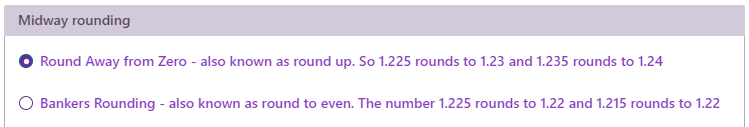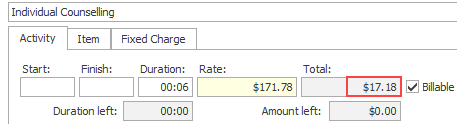# Rounding

This page simplifies discussion by working in Australian dollars. The general principles apply to other currencies.

## Midway rounding

Case Manager stores many values to 4 decimal places. However, when costs and taxes are calculated the results must be expressed in dollars and cents. Often some kind of rounding is required to bring the result to an exact amount of dollars and cents, i.e. to two decimal places.

For example, a cost rate is multiplied by the time and the result is 154.2256. When converting this number to dollars and cents, the number needs to be rounded to two decimal places.

There are two possibilities: \$154.22 or \$154.23. From version 6.3 onwards you can choose the rounding option you require at the System Accounts settings:Essentially, the first option always rounds up. The second, Bankers Rounding, rounds up half the time and rounds down the rest of the time. The term reflects its use for certain banking computations, because the average of all errors is closer to 0.

### Round away from zero - also known as round up

With this option, numbers are always rounded up.

For example 1.225 is rounded to 1.23; and 1.222 is rounded to 1.22.

### Bankers rounding - also known as round to even

With this option, numbers are rounded to the nearest even number. Thus:

• 1.225 is rounded to 1.22 (the nearest even number).
• 1.235 is rounded to 1.24 (the nearest even number)

Of course, 1.222 is still rounded to 1.22 and 1.227 is still rounded up to 1.23.

Banker's rounding is the default setting within Case Manager.

### Where does this option apply?

This applies everywhere Case Manager either displays currency or does a calculation of currency. So it applies to activities (Activity/Item/Fixed Charge), estimate costs, case costs, invoices, adjustments, journals and reports.

Note that you can enter values with four decimal places, for example a rate of 147.8650 per hour. Case Manager retains the four decimal places in all its calculations until the moment when a conversion to currency is required. This rate (e.g. 147.8650) is displayed on screens with two decimal places, rounded according to your Midway Rounding setting.

### Will changing this option affect my existing data?

No, your historical cases and financial data will not be affected. If you choose to change the setting, it will only affect new costs and invoices created after the setting has been changed.

### Which option should you choose if you don't want anything to change?

Select the first option: Round up

Round up, away from zero, is the default option for a new database and was the previous default setting that Case Manager used. However, there were inconsistencies in some areas of rounding which is why we now provide another option for rounding.

## Cost calculations

Three types of activities provide the foundation for costs.

• For an activity cost the total cost is the hourly rate (including GST) multiplied by the time.
• For an item cost the total cost is the item cost (in dollars and cents) multiplied by the quantity.
• For a fixed charge cost the total cost is already an exact amount of dollars and cents.

### Cost and invoice line item rounding

With activity costs, there are fewer rounding issues with total costs (Ex-tax and including tax) when the duration is a multiple of 6 minutes.

For example, 6 minutes = 0.1 hrs, 12 minutes = 0.2 hrs, and so on. With these durations the activity rate can be multiplied by a simple decimal number.

Below 6 minutes represents 0.1 hour. The total cost = 0.1 multiplied by the hourly rate (\$171.78):However, a duration of 9 minutes represents 0.15 hours. When 0.15 is multiplied by 171.78 the result is 25.767‬.

Clearly 25.767 must be rounded to an exact value in dollars and cents, i.e. \$25.77 or \$25.76, depending on the Midway Rounding setting (above).

In summary, to minimise rounding issues, use activity rates that are simple numbers (if possible) and bill in 6 minute intervals.

## GST rounding

When GST is calculated two methods are approved by the ATO.

Case Manager uses the line item approach where GST is calculated per cost line item. To calculate the total GST for an invoice, the GST for each invoice line item is added.

When the value of a line item's GST has more than 2 decimal places, it is rounded to whole cents, depending on the Midway Rounding setting.

For example, a total of \$54.40 is divided by 11 to calculate the GST of 4.945454545454545... This is rounded to 2 decimal places and becomes \$4.95 or \$4.94, depending on the rounding setting.

When calculated using this method the invoice's total GST may not exactly match the value calculated using 1/11th of the invoice total, rounded to two decimal places (and assuming all costs include tax).

## Rounding and Xero exports

When invoices are exported to Xero, Xero recalculates the invoice line item totals and their GST amounts as new, rounded results.

Usually this does not cause any issues. However, the process is susceptible to rounding errors that change the invoice's GST by a very small amount when compared to Case Manager. Mismatch between invoice details in the two systems causes the export to fail.

To avoid this issue use cost durations that are multiples of 6 minutes. Alternatively, you can select the export option that in effect stops Xero from re-calculating invoice data. See Xero export rounding for details.

## Nominal duration

When a new cost is created, its duration is initially set to the corresponding activity's Nominal duration.

As explained above, to minimise rounding issues the Nominal duration should be set to a value that uses 6 minute increments.

## Estimates and rounding

Cost rounding can lead to a potential issue with estimates where it appears that a minute of a certain activity is 'missing'. This is demonstrated using a very simple estimate example at Estimates and rounding.

As is explained, Case Manager has an estimate setting, the Round final cost to match the estimate total (where possible) checkbox, to provide a solution.

## ReturnToWorkSA charge code rounding

ReturnToWorkSA requires that when activities are invoiced their duration per charge code per day must be a multiple of 6 minutes.

Case Manager can perform charge code rounding to assist with this requirement, see ReturnToWorkSA charge code rounding.